# ISEE Middle Level Quantitative : How to find a proportion

## Example Questions

### Example Question #13 : Ratio And Proportion

The distance between Carson and Miller is 260 miles and is represented by four inches on a map. The distance between Carson and Davis is 104 miles.

Which is the greater quantity?

(a) The distance between Carson and Davis on the map

(b)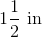(b) is greater

(a) and (b) are equal

(a) is greater

It is impossible to tell from the information given

(a) is greater

Explanation:

Letbe the map distance between Carson and Davis. A proportion statement can be set up relating map inches to real miles: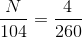Solve for: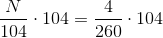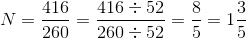Carson and Davis are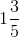inches apart on the map;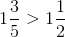### Example Question #14 : Ratio And Proportion

The distance between Vandalia and Clark is 250 miles and is represented by six inches on a map. The distance between Vandalia and Ferrell is represented by three and three-fifths inches on a map.

Which is the greater quantity?

(a) The actual distance between Vandalia and Ferrell

(b) 150 miles

(a) is greater

(b) is greater

(a) and (b) are equal

It is impossible to tell from the information given

(a) and (b) are equal

Explanation:

Letbe the real distance between Vandalia and Ferrell. A proportion statement can be set up relating real miles to map inches: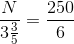Solve for: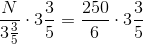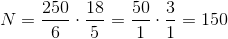The actual distance between Vandalia and Ferrell is 150 miles.

### Example Question #15 : Ratio And Proportion

Jay has a shelf of books, of which 60% are hardback. The rest are paperback. If 12 are hardback, how many paperbacks are there?Explanation:

There are a couple different ways to solve this problem. One way is to set up an equation from the given equation. Essentially, you have to find the total number of books before you can find how many paperbacks. An equation for that could be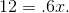In other works, 12 is 60% of what total amount? (Remember, in equations, we convert percentages to decimals.) Then, you would solve for x to get 20 total books. Once you know the total, you can subtract the number of hardbacks from that to get 8 paperbacks. Another way to solve this equation is to set up a proportion. That would be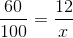. Then, we could cross multiply to get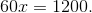Solving for x would again give you 20 and you would repeat the steps from above to get 8.

### Example Question #16 : Ratio And Proportion

A given recipe calls forcups of butter for everycup of flower andcups of sugar. If you wish to triple the recipe, how many total cups of ingredients will you need?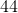Explanation:

This is an easy case of proportions. To triple the recipe, you merely need to triple each of its component parts; therefore, you will have:cups of butter for everycup of flower and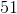cups of sugar

Summing these up, you get: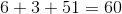total cups.

### Example Question #17 : Ratio And Proportion

A witch's brew containsnewt eyes for everylizard tongues. If Aurelia the witch usednewt eyes in her recipe, how many lizard tongues did she need to use?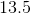Explanation:

To solve this, you need to set up a proportion: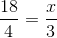Multiply both sides by: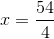Simplifying, this gives you: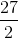orlizard tongues.

### Example Question #18 : Ratio And Proportion

Isidore could buyequally-sized blocks of cheese for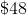. How many could he buy for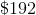?Explanation:

For this problem, set up a proportion: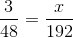, whererepresents the number of cheese blocks that Isidore can buy.

To solve this, multiply both sides by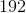: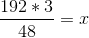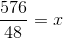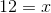### Example Question #19 : Ratio And Proportion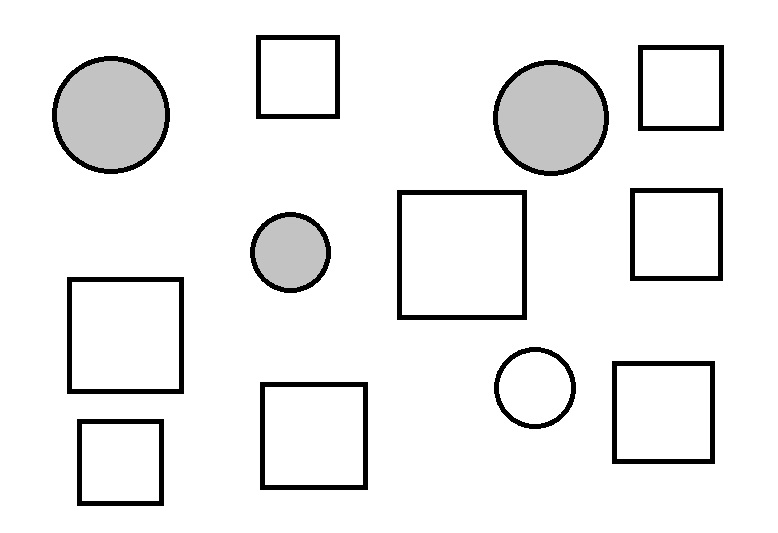Refer to the above diagram. How many squares should be shaded in if it is desired that the fraction of the squares that are shaded in should be equivalent to the fraction of the circles that are shaded in?Explanation:

There are four circles, three of which are shaded; there are eight squares. If we letbe the number of squares to be shaded, then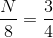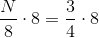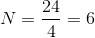### Example Question #20 : Ratio And Proportion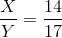Which is the greater quantity?

(a)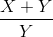(b)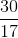It is impossible to determine which quantity is the greater from the information given

(b) is the greater quantity

(a) is the greater quantity

(a) and (b) are equal

(a) is the greater quantity

Explanation:

From a property of proportions, if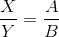, it follows that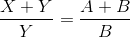. Setting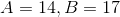,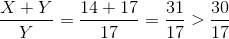.

### Example Question #1 : How To Find A Proportion

A witch's brew containsnewt eyes for everylizard tongues. If Aurelia the witch usednewt eyes in her recipe, how many lizard tongues did she need to use?Explanation:

To solve this, you need to set up a proportion:Multiply both sides by:Simplifying, this gives you:orlizard tongues.